# Check if given sorted subsequence exits in the Binary Search Tree or Not

In this article, we are going to see how we can check whether a given sorted subsequence exists in a given Binary Search Tree or Not?
Submitted by Radib Kar, on October 31, 2020

Here, we are going to discuss another application of inorder traversal in BST. We know that inorder traversal in a Binary search tree produces a sorted list of the keys in the BST. Using that fact, we can check whether a given sorted subsequence exists in the Binary Search Tree or not. Say, the given Binary search tree is the below one: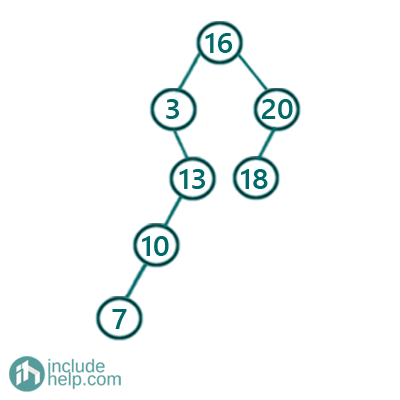Say, we have given two sequences to check where they exist or not.

Sequence 1: [7, 13, 18, 20]

Sequence 2: [7, 13, 14, 20]

The first sequence is there in the given Binary search tree, but the second one is not. So How we can solve that with inorder traversal? We already know that inorder traversal in a Binary search tree produces a sorted list. So we can use that fact and create the sorted list and then just search for the sequence elements one by one which is linear in run time but needs extra storage. So instead of that without storing the inorder traversal, we can check the sequence elements one by one while doing the inorder traversal.

The idea is that we will keep an index pointer to the first element of the sequence and while doing the inorder traversal while we find that indexed element we increment the index pointer. After doing the inorder traversal if we find that the index pointer has reached the end of the sequence, i.e., the entire sequence is in the Binary search tree, otherwise, it's not.

Below is the visualization of the above idea.

So the input BST is the same as the example one and sequence is the first one, [7, 13, 18, 20]

So the inorder traversal starts from the root and the first node visited is 3 and then it visits 7 which is the first element of the sequence too. So increment the index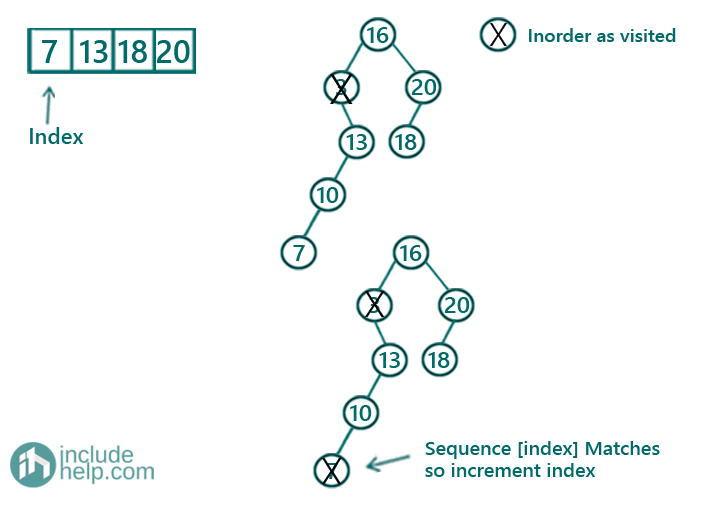Then it visits 10 and after that 13. Again it's the current element in the sequence, so increment the index.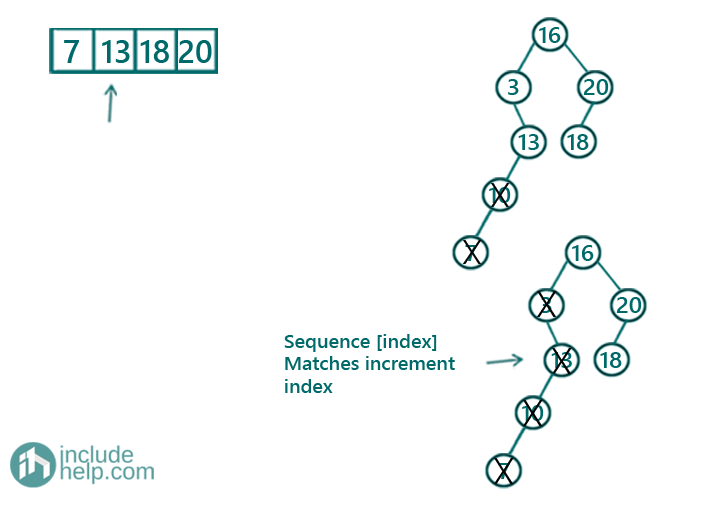Doing the same, below are the steps till entire inorder traversal finishes.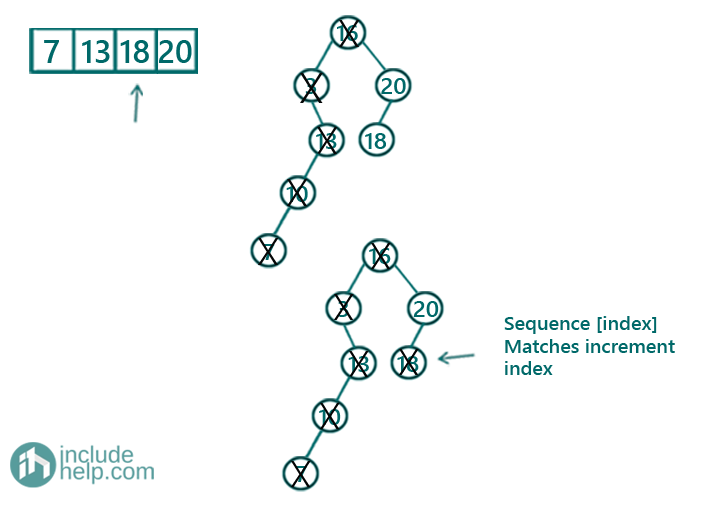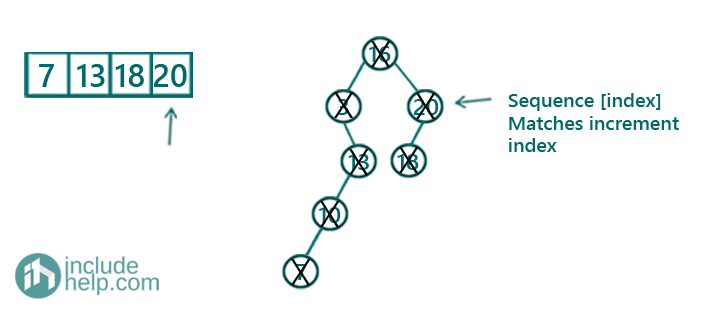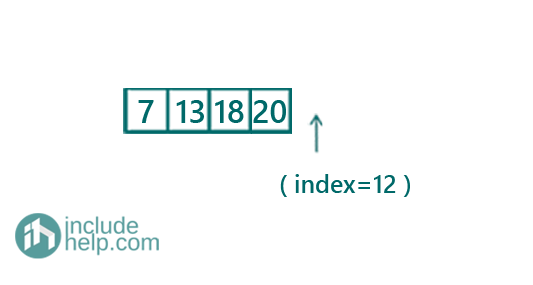So after the inorder traversal finishes, the index pointer reaches the end of the sequence, hence, the sequence is present in the Binary search tree.

For the sequence 2, when the index pointer reaches at 14, it doesn't get incremented further since 14 isn't found in the BST.

Below is the C++ implementation for the above idea.

```#include <bits/stdc++.h>
using namespace std;

// tree node is defined
class TreeNode {
public:
int val;
TreeNode* left;
TreeNode* right;
TreeNode(int data)
{
val = data;
left = NULL;
right = NULL;
}
};

// inorder traversal to check if the given
// sorted subsequence exists in the BST
void DoesSeqExistRec(TreeNode* root, vector<int> sequence, int& index)
{
//base case
if (!root)
return;

// traverse left subtree first as it's inorder traversal
DoesSeqExistRec(root->left, sequence, index);

// If current node matches with current sequence
// element(sequence[index]) then move
// to the next element in the sequenece
if (root->val == sequence[index])
index++;

// traverse right subtree
DoesSeqExistRec(root->right, sequence, index);
}

// function to check whether the sequence exists or not
bool DoesSeqExist(TreeNode* root, vector<int> sequence)
{

int index = 0;

// recursive function to check if all elements
// of the sequence are in the BST or not
DoesSeqExistRec(root, sequence, index);

//if after the inorder traversal as we have discussed,
//index reaches towards the end
//then the BST contains the sequence other wise not
if (index == sequence.size())
return true;
else
return false;
}

int main()
{
TreeNode* root = new TreeNode(16);

root->left = new TreeNode(3);
root->left->right = new TreeNode(13);
root->left->right->left = new TreeNode(10);
root->left->right->left->left = new TreeNode(7);
root->right = new TreeNode(20);
root->right->left = new TreeNode(18);

vector<int> sequence1{ 7, 13, 18, 20 };

if (DoesSeqExist(root, sequence1))
cout << "Yes the sequence exists in the binary search tree\n";
else
cout << "No, the sequence exists in the binary search tree";

vector<int> sequence2{ 7, 13, 14, 20 };

if (DoesSeqExist(root, sequence2))
cout << "Yes the sequence exists in the binary search tree\n";
else
cout << "No, the sequence doesn't exist in the binary search tree";

return 0;
}
```

Output:

```Yes the sequence exists in the binary search tree
No, the sequence doesn't exist in the binary search tree
```

Languages: » C » C++ » C++ STL » Java » Data Structure » C#.Net » Android » Kotlin » SQL
Web Technologies: » PHP » Python » JavaScript » CSS » Ajax » Node.js » Web programming/HTML
Solved programs: » C » C++ » DS » Java » C#
Aptitude que. & ans.: » C » C++ » Java » DBMS
Interview que. & ans.: » C » Embedded C » Java » SEO » HR
CS Subjects: » CS Basics » O.S. » Networks » DBMS » Embedded Systems » Cloud Computing
» Machine learning » CS Organizations » Linux » DOS
More: » Articles » Puzzles » News/Updates